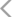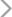• 综合
• 股票
• 板块
• 嘉宾
• 课程
• 基金
• 经理
• 说说
• 掌评
• 消息冯杰

VIP2017-12-07 09:41:57 展开全文 互动详情 470人气

2016-09-28 15:20:30 展开全文 互动详情 517人气
DRAWTEXT(T24=1 AND CURRBARSCOUNT=58,AAA-(AAA*TS24),'上升三部曲,上涨信号‖'),COLORFF00FF  ;
DRAWTEXT(T25=1 AND CURRBARSCOUNT=58,AAA-(AAA*TS25),'下跌三部曲,下跌信号‖'),COLORFFFF00  ;
DRAWTEXT(T26=1 AND CURRBARSCOUNT=58,AAA-(AAA*TS26),'跳空缺口,突破盘整,向上涨、向下跌开始‖'),COLOR00FFFF  ;
DRAWTEXT(T27=1 AND CURRBARSCOUNT=58,AAA-(AAA*TS27),'三只乌鸦站枝头,可能见顶回落‖'),COLORFFFF00  ;
DRAWTEXT(T28=1 AND CURRBARSCOUNT=58,AAA-(AAA*TS28),'光脚阴线,下跌信号‖'),COLORFFFF00  ;
DRAWTEXT(T29=1 AND CURRBARSCOUNT=58,AAA-(AAA*TS29),'光头阳线,多方积极上攻态势,后市看涨‖'),COLOR0000FF  ;
DRAWTEXT(T30=1 AND CURRBARSCOUNT=58,AAA-(AAA*TS30),'分离,注意看涨分离和看跌分离‖'),COLOR00FFFF  ;
DRAWTEXT(T31=1 AND CURRBARSCOUNT=58,AAA-(AAA*TS31),'长下影,有可能止跌回升,升势末期,须小心‖'),COLOR00FFFF  ;
DRAWTEXT(T32=1 AND CURRBARSCOUNT=58,AAA-(AAA*TS32),'长上影,表明行情上档压力沉重,升势受阻‖'),COLORFFFF00  ;
DRAWTEXT(T33=1 AND CURRBARSCOUNT=58,AAA-(AAA*TS33),'十字星,重要的警告信号,原趋势停顿或反转‖'),COLOR00FFFF  ;
DRAWTEXT(T34=1 AND CURRBARSCOUNT=58,AAA-(AAA*TS34),'大阴烛,后市向淡,发生逆转‖'),COLORFF0000  ;
DRAWTEXT(T35=1 AND CURRBARSCOUNT=58,AAA-(AAA*TS35),'好友反攻,跌势已尽,要展开一段反弹行情‖'),COLORFF00FF  ;
DRAWTEXT(T36=1 AND CURRBARSCOUNT=58,AAA-(AAA*TS36),'倾盆大雨,见顶信号‖'),COLORFFFF00  ;
DRAWTEXT(T37=1 AND CURRBARSCOUNT=58,AAA-(AAA*TS37),'今日成交量大于昨日,且有继续增大趋势'),COLORYELLOW ;
DRAWTEXT(T38=1 AND CURRBARSCOUNT=58,AAA-(AAA*TS38),'今日成交量小于昨日，但有放大迹象'),COLORFFFF00  ;
DRAWTEXT(T39=1 AND CURRBARSCOUNT=58,AAA-(AAA*TS39),'今日成交量大于昨日, 但有缩小迹象'),COLORRED  ;
DRAWTEXT(T40=1 AND CURRBARSCOUNT=58,AAA-(AAA*TS40),'今日成交量小于昨日，且有缩小迹象'),COLORRED   ;
DRAWTEXT(T41=1 AND CURRBARSCOUNT=58,AAA-(AAA*TS41),'极端行情,在未来的几日内有八成的机会变更方向'),COLORWHITE ;
DRAWTEXT(T42=1 AND CURRBARSCOUNT=58,AAA-(AAA*TS42),'上涨趋势'),COLORRED  ;
DRAWTEXT(T43=1 AND CURRBARSCOUNT=58,AAA-(AAA*TS43),'下跌趋势'),COLORGREEN;
2016-09-28 15:18:23 展开全文 互动详情 424人气
DRAWTEXT(T1=1 AND CURRBARSCOUNT=58,AAA-(AAA*TS1),'早晨之星,见底回升‖'),COLORFF00FF  ;
DRAWTEXT(T2=1 AND CURRBARSCOUNT=58,AAA-(AAA*TS2),'黄昏之星,见顶回落‖'),COLORFFFF00  ;
DRAWTEXT(T3=1 AND CURRBARSCOUNT=58,AAA-(AAA*TS3),'长十字,重要的警告信号,原趋势停顿或反转‖'),COLOR00FFFF  ;
DRAWTEXT(T4=1 AND CURRBARSCOUNT=58,AAA-(AAA*TS4),'垂死十字,下跌信号‖'),COLORFFFF00  ;
DRAWTEXT(T5=1 AND CURRBARSCOUNT=58,AAA-(AAA*TS5),'早晨十字星,上涨信号,见底回升‖'),COLORFF00FF  ;
DRAWTEXT(T6=1 AND CURRBARSCOUNT=58,AAA-(AAA*TS6),'黄昏十字星,趋势停顿或反转,下跌或,见顶回落‖'),COLORFFFF00  ;
DRAWTEXT(T7=1 AND CURRBARSCOUNT=58,AAA-(AAA*TS7),'射击之星,市场顶部就在眼前,可能见顶回落,可靠性低‖'),COLORFFFF00  ;
DRAWTEXT(T8=1 AND CURRBARSCOUNT=58,AAA-(AAA*TS8),'倒转锤头构成一个看涨信号,可能见底回升‖'),COLORFF00FF  ;
DRAWTEXT(T9=1 AND CURRBARSCOUNT=58,AAA-(AAA*TS9),'锤头,可能见底回升,如有量配合,信号强烈‖'),COLOR0000FF  ;
DRAWTEXT(T10=1 AND CURRBARSCOUNT=58,AAA-(AAA*TS10),'吊颈,上升行情中见顶回落,顶部出现,见顶信号‖'),COLORFFFF00  ;
DRAWTEXT(T11=1 AND CURRBARSCOUNT=58,AAA-(AAA*TS11),'穿头破脚,顶部出现,见顶回落信号‖'),COLORFFFF00  ;
DRAWTEXT(T12=1 AND CURRBARSCOUNT=58,AAA-(AAA*TS12),'乌云盖顶,见顶回落信号‖'),COLORFFFF00  ;
DRAWTEXT(T13=1 AND CURRBARSCOUNT=58,AAA-(AAA*TS13),'曙光初现,将演化为V型反转或横盘。后市见底回升‖'),COLORFF00FF  ;
DRAWTEXT(T14=1 AND CURRBARSCOUNT=58,AAA-(AAA*TS14),'身怀六甲,底部,是见底回升,顶部,是见顶回落信号‖'),COLOR00FFFF  ;
DRAWTEXT(T15=1 AND CURRBARSCOUNT=58,AAA-(AAA*TS15),'十字胎‖'),COLOR00FFFF  ;
DRAWTEXT(T16=1 AND CURRBARSCOUNT=58,AAA-(AAA*TS16),'平顶,行情见顶,股价将反转。但下跌力度较小‖'),COLORFFFFFF  ;
DRAWTEXT(T17=1 AND CURRBARSCOUNT=58,AAA-(AAA*TS17),'平底,行情探低见底,股价行将反转上升,但力度较小‖'),COLORFFFFFF  ;
DRAWTEXT(T18=1 AND CURRBARSCOUNT=58,AAA-(AAA*TS18),'大阳烛,上升中,剧烈地向上攀升。下行中,剧烈地向上反弹‖'),COLOR0000FF  ;
DRAWTEXT(T19=1 AND CURRBARSCOUNT=58,AAA-(AAA*TS19),'三个白武士,每日收盘价上移,表示可能见底回升‖'),COLORFFFFFF  ;
DRAWTEXT(T20=1 AND CURRBARSCOUNT=58,AAA-(AAA*TS20),'双飞乌鸦,行情开始疲软将见顶回落‖'),COLORFF0000  ;
DRAWTEXT(T21=1 AND CURRBARSCOUNT=58,AAA-(AAA*TS21),'孕育线,注意股价位置‖'),COLOR00FFFF  ;
DRAWTEXT(T22=1 AND CURRBARSCOUNT=58,AAA-(AAA*TS22),'多方炮,底部可能上涨,中间上涨中继,顶部见顶信号‖'),COLOR00FFFF  ;
DRAWTEXT(T23=1 AND CURRBARSCOUNT=58,AAA-(AAA*TS23),'出水芙蓉,阳线穿过20、40、60日均线,上攻势头初现‖'),COLORFF00FF  ;
2016-09-28 15:17:44 展开全文 互动详情 352人气
TS1:=IF(T1=1,0+0.03,0);
TS2:=IF(T2=1,TS1+0.03,TS1);
TS3:=IF(T3=1,TS2+0.03,TS2);
TS4:=IF(T4=1,TS3+0.03,TS3);
TS5:=IF(T5=1,TS4+0.03,TS4);
TS6:=IF(T6=1,TS5+0.03,TS5);
TS7:=IF(T7=1,TS6+0.03,TS6);
TS8:=IF(T8=1,TS7+0.03,TS7);
TS9:=IF(T9=1,TS8+0.03,TS8);
TS10:=IF(T10=1,TS9+0.03,TS9);
TS11:=IF(T11=1,TS10+0.03,TS10);
TS12:=IF(T12=1,TS11+0.03,TS11);
TS13:=IF(T13=1,TS12+0.03,TS12);
TS14:=IF(T14=1,TS13+0.03,TS13);
TS15:=IF(T15=1,TS14+0.03,TS14);
TS16:=IF(T16=1,TS15+0.03,TS15);
TS17:=IF(T17=1,TS16+0.03,TS16);
TS18:=IF(T18=1,TS17+0.03,TS17);
TS19:=IF(T19=1,TS18+0.03,TS18);
TS20:=IF(T20=1,TS19+0.03,TS19);
TS21:=IF(T21=1,TS20+0.03,TS20);
TS22:=IF(T22=1,TS21+0.03,TS21);
TS23:=IF(T23=1,TS22+0.03,TS22);
TS24:=IF(T24=1,TS23+0.03,TS23);
TS25:=IF(T25=1,TS24+0.03,TS24);
TS26:=IF(T26=1,TS25+0.03,TS25);
TS27:=IF(T27=1,TS26+0.03,TS26);
TS28:=IF(T28=1,TS27+0.03,TS27);
TS29:=IF(T29=1,TS28+0.03,TS28);
TS30:=IF(T30=1,TS29+0.03,TS29);
TS31:=IF(T31=1,TS30+0.03,TS30);
TS32:=IF(T32=1,TS31+0.03,TS31);
TS33:=IF(T33=1,TS32+0.03,TS32);
TS34:=IF(T34=1,TS33+0.03,TS33);
TS35:=IF(T35=1,TS34+0.03,TS34);
TS36:=IF(T36=1,TS35+0.03,TS35);
TS37:=IF(T37=1,TS36+0.03,TS36);
TS38:=IF(T38=1,TS37+0.03,TS37);
TS39:=IF(T39=1,TS38+0.03,TS38);
TS40:=IF(T40=1,TS39+0.03,TS39);
TS41:=IF(T41=1,TS40+0.03,TS40);
TS42:=IF(T42=1,TS41+0.03,TS41);
TS43:=IF(T43=1,TS42+0.03,TS42);
2016-09-28 15:17:04 展开全文 互动详情 306人气
AND REF(LOW,4)<REF(LOW,3) AND REF(LOW,4)<REF(LOW,2) AND REF(LOW,4)
<REF(LOW,1) AND REF(HIGH,4)>REF(HIGH,3) AND REF(HIGH,4)>REF(HIGH,2) AND REF(HIGH,4)>REF(HIGH,1) AND CLOSE/OPEN<0.97 AND
CLOSE<REF(CLOSE,4)),1,0));
T26{跳空缺口}:=CONST(IF(HIGH<REF(LOW,1) OR LOW>REF(HIGH,1),1,0));
T27{三只乌鸦}:=CONST(IF((REF(CLOSE,2)<REF(OPEN,2) AND REF(CLOSE,1)
<REF(OPEN,1) AND CLOSE<OPEN AND REF(CLOSE,1)<REF(CLOSE,2)
AND CLOSE<REF(CLOSE,1)),1,0));
T28{光脚阴线}:=CONST(IF((LOW=CLOSE AND HIGH!=LOW),1,0));
T29{光头阳线}:=CONST(IF((HIGH=CLOSE AND HIGH!=LOW),1,0));
T30{分离}:=CONST(IF((OPEN=REF(OPEN,1) AND (CLOSE-OPEN)*(REF(CLOSE,1)
-REF(OPEN,1))<0),1,0));
T31{长下影}:=CONST(IF(((MIN(CLOSE,OPEN)-LOW)/(HIGH-LOW)>0.667),1,0));
T32{长上影}:=CONST(IF(((HIGH-MAX(CLOSE,OPEN))/(HIGH-LOW)>0.667),1,0));
T33{十字星}:=CONST(IF((CLOSE=OPEN AND HIGH!=LOW),1,0));
T34{大阴烛}:=CONST(IF((OPEN/CLOSE > 1.05 AND HIGH/LOW < OPEN/CLOSE+0.018),1,0));
T35{好友反攻}:=CONST(IF(((REF(CLOSE,1)<REF(OPEN,1) AND CLOSE>OPEN
AND ABS(CLOSE-REF(CLOSE,1))/CLOSE<0.002) OR (REF(CLOSE,1)>REF(OPEN,1)
AND CLOSE<OPEN AND ABS(CLOSE-REF(CLOSE,1))
/CLOSE<0.002)),1,0));
T36{倾盆大雨}:=CONST(IF((REF(C,1)/REF(O,1)>=1.03 AND O<REF(C,1) AND O
>REF(O,1) AND C<REF(O,1)),1,0));
T37{M9}:=CONST(V*比>REF(V,1) AND MA(V,5)>REF(MA(V,5),1));
T38{M10}:=CONST(V*比<REF(V,1) AND MA(V,5)>REF(MA(V,5),1));
T39{M11}:=CONST(V*比>REF(V,1)AND MA(V,5)<REF(MA(V,5),1));
T40{M12}:=CONST(V*比<REF(V,1)AND MA(V,5)<REF(MA(V,5),1));
T41{M13}:=CONST((((IF((CLOSE = OPEN),1,0) + IF((CLOSE >= J12),1,0)) + IF((CLOSE <= J23),1,0)) > 1));
T42{M14}:=CONST((((AMOUNT / VOL) / 100) > REF(HIGH,1)));
T43{M15}:=CONST((((AMOUNT / VOL) / 100) < REF(LOW,1)));
2016-09-28 15:16:28 展开全文 互动详情 312人气
AND ABS(CLOSE-OPEN)/CLOSE<0.005 AND
MAX(CLOSE,OPEN) < MAX(REF(CLOSE,1),REF(OPEN,1)) AND MIN(CLOSE,OPEN)
> MIN(REF(CLOSE,1),REF(OPEN,1))),1,0));
T15{十字胎}:=CONST(IF((ABS(REF(CLOSE,1)-REF(OPEN,1))/REF(CLOSE,1) > 0.04
AND CLOSE=OPEN AND
CLOSE < MAX(REF(CLOSE,1),REF(OPEN,1)) AND CLOSE > MIN(REF(CLOSE,1)
,REF(OPEN,1))),1,0));
T16{平顶}:=CONST(IF((ABS(HIGH-REF(HIGH,1))/HIGH<0.001),1,0));
T17{平底}:=CONST(IF(((ABS(LOW-REF(LOW,1))/LOW<0.001 AND ABS(REF(LOW,1)
-REF(LOW,2))/REF(LOW,1)<=0.001)),1,0));
T18{大阳烛}:=CONST(IF((CLOSE/OPEN>1.05 AND HIGH/LOW < CLOSE/OPEN+0.018),1,0));
T19{三个白武士}:=CONST(IF((REF(CLOSE,2)>REF(OPEN,2) AND REF(CLOSE,1)
>REF(OPEN,1) AND CLOSE>OPEN
AND REF(CLOSE,1)>REF(CLOSE,2) AND CLOSE>REF(CLOSE,1)),1,0));
T20{双飞乌鸦}:=CONST(IF((REF(CLOSE,1)<REF(OPEN,1) AND CLOSE<OPEN
AND CLOSE/OPEN<0.98),1,0));
T21{孕育线}:=CONST(IF((REF(CLOSE,2)>REF(OPEN,2) AND (REF(CLOSE,2)
-REF(OPEN,2))/REF(OPEN,2)>=2/100  AND REF(OPEN,1)>=REF(CLOSE,1)
AND (REF(OPEN,1)-REF(CLOSE,1))/REF(CLOSE,1)<=2/100 AND REF(OPEN,1)
<=REF(CLOSE,2) AND REF(CLOSE,1)>=REF(OPEN,2) AND C>=OPEN
AND (CLOSE-OPEN)/OPEN>=2/100 AND CLOSE>=REF(OPEN,1) AND OPEN<=REF(CLOSE,1)
AND (MAX(CLOSE,REF(C,2))-MIN(CLOSE,REF(CLOSE,2)))/MIN(CLOSE,REF(CLOSE,2))
<=1/100),1,0));
T22{多方炮}:=CONST(IF((REF(CLOSE,2)>REF(OPEN,2) AND REF(CLOSE,1)<REF(OPEN,1)
AND CLOSE>OPEN),1,0));
T23{出水芙蓉}:=CONST(IF(((OPEN<EMA(C,20) OR OPEN<EMA(C,40) OR OPEN<
EMA(C,60)) AND (C>MAX(EMA(C,20),MAX(EMA(C,40),EMA(C,60))))AND (V/MA(V,30)
>1.2 AND C/REF(C,1)>1.049)),1,0));
T24{上升三部曲}:=CONST(IF((REF(CLOSE,4)/REF(OPEN,4)>1.03 AND REF(CLOSE,3)
<REF(OPEN,3) AND REF(CLOSE,2)<REF(OPEN,2) AND REF(CLOSE,1)<REF(OPEN,1)
AND REF(LOW,4)<REF(LOW,3) AND REF(LOW,4)<REF(LOW,2) AND REF(LOW,4)
<REF(LOW,1) AND REF(HIGH,4)>REF(HIGH,3) AND REF(HIGH,4)>REF(HIGH,2)
AND REF(HIGH,4)>REF(HIGH,1) AND CLOSE/OPEN>1.03 AND CLOSE>REF(CLOSE,4)),1,0));
T25{下跌三部曲}:=CONST(IF(( REF(CLOSE,4)/REF(OPEN,4)<0.97 AND REF(CLOSE,3)
>REF(OPEN,3) AND REF(CLOSE,2)>REF(OPEN,2) AND REF(CLOSE,1)>REF(OPEN,1)
2016-09-28 15:15:04 展开全文 互动详情 247人气
TT:=240/FROMOPEN;

J12:=(HIGH - ((HIGH - LOW) / 3));
J23:=(LOW + ((HIGH - LOW) / 3));
AAA:=CONST(HHV(H,90));
BBB:=CONST(LLV(L,100));

T1{早晨之星}:=CONST(IF((REF(CLOSE,2)/REF(OPEN,2) < 0.95 AND REF(OPEN,1)
< REF(CLOSE,2) AND ABS(REF(OPEN,1)-REF(CLOSE,1))/REF(CLOSE,1)<0.03 AND
CLOSE/OPEN>1.05 AND CLOSE>REF(CLOSE,2)),1,0));
T2{黄昏之星}:=CONST(IF((REF(CLOSE,2)/REF(OPEN,2) > 1.03 AND REF(OPEN,1)
> REF(CLOSE,2) AND ABS(REF(OPEN,1)-REF(CLOSE,1))/REF(CLOSE,1)<0.02
AND CLOSE/OPEN<0.97 AND CLOSE<REF(CLOSE,2)),1,0));
T3{长十字}:=CONST(IF((CLOSE=OPEN AND HIGH/LOW >1.03),1,0));
T4{垂死十字}:=CONST(IF((CLOSE=OPEN AND CLOSE=LOW AND CLOSE!=HIGH),1,0));
T5{早晨十字星}:=CONST(IF((REF(CLOSE,2)/REF(OPEN,2) < 0.95 AND REF(OPEN,1)
< REF(CLOSE,2) AND REF(OPEN,1)=REF(CLOSE,1) AND
CLOSE/OPEN>1.05 AND CLOSE>REF(CLOSE,2)),1,0));
T6{黄昏十字星}:=CONST(IF((REF(CLOSE,2)/REF(OPEN,2) > 1.05 AND REF(OPEN,1)
> REF(CLOSE,2) AND REF(OPEN,1)=REF(CLOSE,1) AND
CLOSE/OPEN<0.95 AND CLOSE<REF(CLOSE,2)),1,0));
T7{射击之星}:=CONST(IF((MIN(OPEN,CLOSE)=LOW AND HIGH-LOW >
3*(MAX(OPEN,CLOSE)-LOW) AND CLOSE > MA(CLOSE,5)),1,0));
T8{倒转锤头}:=CONST(IF((MIN(OPEN,CLOSE)=LOW AND HIGH-LOW >
3*(MAX(OPEN,CLOSE)-LOW) AND CLOSE < MA(CLOSE,5)),1,0));
T9{锤头}:=CONST(IF((HIGH = MAX(OPEN,CLOSE) AND HIGH-LOW >
3*(HIGH-MIN(OPEN,CLOSE)) AND CLOSE < MA(CLOSE,5)),1,0));
T10{吊颈}:=CONST(IF((HIGH = MAX(OPEN,CLOSE) AND HIGH-LOW >
3*(HIGH-MIN(OPEN,CLOSE)) AND CLOSE>MA(CLOSE,5)),1,0));
T11{穿头破脚}:=CONST(IF(((REF(CLOSE,1)/REF(OPEN,1)>1.03 AND CLOSE/OPEN<0.96
AND CLOSE<REF(OPEN,1)
AND OPEN>REF(CLOSE,1))OR (REF(CLOSE,1)/REF(OPEN,1)<0.97 AND CLOSE/OPEN>1.04
AND CLOSE>REF(OPEN,1) AND OPEN<REF(CLOSE,1))),1,0));
T12{乌云盖顶}:=CONST(IF((REF(CLOSE,1)/REF(OPEN,1)>1.03 AND CLOSE/OPEN<0.97
AND OPEN>REF(CLOSE,1) AND CLOSE<REF(CLOSE,1)),1,0));
T13{曙光初现}:=CONST(IF((REF(CLOSE,1)/REF(OPEN,1)<0.97 AND CLOSE/OPEN>1.03
AND OPEN<REF(CLOSE,1) AND CLOSE>REF(CLOSE,1)),1,0));
T14{身怀六甲}:=CONST(IF((ABS(REF(CLOSE,1)-REF(OPEN,1))/REF(CLOSE,1) > 0.04
AND ABS(CLOSE-OPEN)/CLOSE<0.005 AND
MAX(CLOSE,
2016-09-28 15:14:02 展开全文 互动详情 299人气

TT:=240/FROMOPEN;

J12:=(HIGH - ((HIGH - LOW) / 3));

J23:=(LOW + ((HIGH - LOW) / 3));

AAA:=CONST(HHV(H,90));

BBB:=CONST(LLV(L,100));

T1{早晨之星}:=CONST(IF((REF(CLOSE,2)/REF(OPEN,2) < 0.95 AND REF(OPEN,1)

< REF(CLOSE,2) AND ABS(REF(OPEN,1)-REF(CLOSE,1))/REF(CLOSE,1)<0.03 AND

CLOSE/OPEN>1.05 AND CLOSE>REF(CLOSE,2)),1,0));

T2{黄昏之星}:=CONST(IF((REF(CLOSE,2)/REF(OPEN,2) > 1.03 AND REF(OPEN,1)

> REF(CLOSE,2) AND ABS(REF(OPEN,1)-REF(CLOSE,1))/REF(CLOSE,1)<0.02

AND CLOSE/OPEN<0.97 AND CLOSE<REF(CLOSE,2)),1,0));

T3{长十字}:=CONST(IF((CLOSE=OPEN AND HIGH/LOW >1.03),1,0));

T4{垂死十字}:=CONST(IF((CLOSE=OPEN AND CLOSE=LOW AND CLOSE!=HIGH),1,0));

T5{早晨十字星}:=CONST(IF((REF(CLOSE,2)/REF(OPEN,2) < 0.95 AND REF(OPEN,1)

< REF(CLOSE,2) AND REF(OPEN,1)=REF(CLOSE,1) AND

CLOSE/OPEN>1.05 AND CLOSE>REF(CLOSE,2)),1,0));

T6{黄昏十字星}:=CONST(IF((REF(CLOSE,2)/REF(OPEN,2) > 1.05 AND REF(OPEN,1)

> REF(CLOSE,2) AND REF(OPEN,1)=REF(CLOSE,1) AND

CLOSE/OPEN<0.95 AND CLOSE<REF(CLOSE,2)),1,0));

T7{射击之星}:=CONST(IF((MIN(OPEN,CLOSE)=LOW AND HIGH-LOW >

3*(MAX(OPEN,CLOSE)-LOW) AND CLOSE > MA(CLOSE,5)),1,0));

T8{倒转锤头}:=CONST(IF((MIN(OPEN,CLOSE)=LOW AND HIGH-LOW >

3*(MAX(OPEN,CLOSE)-LOW) AND CLOSE < MA(CLOSE,5)),1,0));

T9{锤头}:=CONST(IF((HIGH = MAX(OPEN,CLOSE) AND HIGH-LOW >

3*(HIGH-MIN(OPEN,CLOSE)) AND CLOSE < MA(CLOSE,5)),1,0));

T10{吊颈}:=CONST(IF((HIGH = MAX(OPEN,CLOSE) AND HIGH-LOW >

3*(HIGH-MIN(OPEN,CLOSE)) AND CLOSE>MA(CLOSE,5)),1,0));

T11{穿头破脚}:=CONST(IF(((REF(CLOSE,1)/REF(OPEN,1)>1.03 AND CLOSE/OPEN<0.96

AND CLOSE<REF(OPEN,1)

AND OPEN>REF(CLOSE,1))OR (REF(CLOSE,1)/REF(OPEN,1)<0.97 AND CLOSE/OPEN>1.04

AND CLOSE>REF(OPEN,1) AND OPEN<REF(CLOSE,1))),1,0));

T12{乌云盖顶}:=CONST(IF((REF(CLOSE,1)/REF(OPEN,1)>1.03 AND CLOSE/OPEN<0.97

AND OPEN>REF(CLOSE,1) AND CLOSE<REF(CLOSE,1)),1,0));

T13{曙光初现}:=CONST(IF((REF(CLOSE,1)/REF(OPEN,1)<0.97 AND CLOSE/OPEN>1.03

AND OPEN<REF(CLOSE,1) AND CLOSE>REF(CLOSE,1)),1,0));

T14{身怀六甲}:=CONST(IF((ABS(REF(CLOSE,1)-REF(OPEN,1))/REF(CLOSE,1) > 0.04

AND ABS(CLOSE-OPEN)/CLOSE<0.005 AND

MAX(CLOSE,

2016-09-28 13:15:24 展开全文 互动详情 534人气

1．白色的短期 RSI值在２０以下,由下向上交叉黄色的长期RSI值时为买入信号。

2．白色的短期RSI值在８０以上,由上向下交叉黄色的长期RSI值时为卖出信号。

3．短期 RSI值由上向下突破５０,代表股价已经转弱。

4．短期 RSI值由下向上突破５０,代表股价已经转强。

5．当 RSI值高于８０进入超买区,股价随时可能形成短期回档。

6．当 RSI值低于２０进入超卖区,股价随时可能形成短期反弹。

7．股价一波比一波高,而ＲＳＩ一波比一波低。形成顶背离,行情可能反转下跌。用 RSI判断底部图形较不明显。

8．将 RSI的两个连续低点Ａ、Ｂ连成一条直线,当 RSI向下跌破这条线时,为卖出信号。

9．将RSI的两个连续峰顶Ｃ、Ｄ连成一条直线,当 RSI向上突破这条线时,为买入信号。

10，当RSI1金叉RSI2，并在同一天上80。可以作为追涨依据，如当天放出天量，追涨的成功率更高。可以在选股器里设定好选股条件，快速选出股票。

这是最后的疯狂，也是冲顶的信号，此时是涨幅最大的一波，也是风险最大的时候！所以一定要快进快出，第二天涨停封不住就跑，同时一定要设止损位。打不赢就跑！

2016-05-16 10:09:13 展开全文 互动详情 622人气

实盘数据点掌实盘说明

• 点掌主题
• 点掌成长
• 点掌蓝筹

• 一周
• 一月
• 三月
• 六月
• 一年
• 创建以来
• 对标涨跌：• 手机号码：

请输入手机号码，您的个人信息严格保密，请放心

• 图形验证码：

请输入右侧图形验证码

• 短信验证码：

请输入接收的短信验证码

获取短信验证码
X

X

（一）按照“后台实名、前台自愿”原则，对注册用户进行真实身份信息认证，不得向未认证真实身份信息的用户提供跟帖评论服务。

（二）建立健全用户信息保护制度，收集、使用用户个人信息应当遵循合法、正当、必要的原则，公开收集、使用规则，明示收集、使用信息的目的、方式和范围，并经被收集者同意。

（三）对新闻信息提供跟帖评论服务的，应当建立先审后发制度。

（四）提供“弹幕”方式跟帖评论服务的，应当在同一平台和页面同时提供与之对应的静态版信息内容。

（五）建立健全跟帖评论审核管理、实时巡查、应急处置等信息安全管理制度，及时发现和处置违法信息，并向有关主管部门报告。

（六）开发跟帖评论信息安全保护和管理技术，创新跟帖评论管理方式，研发使用反垃圾信息管理系统，提升垃圾信息处置能力；及时发现跟帖评论服务存在的安全缺陷、漏洞等风险，采取补救措施，并向有关主管部门报告。

（七）配备与服务规模相适应的审核编辑队伍，提高审核编辑人员专业素养。

（八）配合有关主管部门依法开展监督检查工作，提供必要的技术、资料和数据支持。• 10牛
• 30牛
• 50牛
• 其它